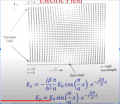# exponent turns field into zero amplitude

#### yef smith

Joined Aug 2, 2020
293
Hello,bellow we can see the field lines and its formula's.
for x=a/2 and z=lambda/4 we suppose to get Ex=0 Ez=Max
from E_x the cosine is zero
but E_z is zero also because the exponent will give us complex "j"
in both Ex and Ez there are exponent expressions with z variable.
How mathematickly this exponent can cause the whole expression to be zero?(as shown in the field lines bellow)
Thanks.